# 【matplotlib基础】--几何图形

matplitlib 中的 patches 类提供了丰富的几何对象，

# 1. 多边形类

## 1.1. 矩形

import matplotlib.pyplot as plt
import matplotlib.patches as mptch

fig = plt.figure(figsize=[6, 6])

r1 = mptch.Rectangle(xy=(0.2, 0.3),
height=0.5,
width=0.7,
color="lightblue")
r2 = mptch.Rectangle(xy=(0.4, 0.6),
height=0.3,
width=0.2,
angle=60,
color="lightgreen")

plt.show()


Rectangle对象的主要参数

1. xy：矩形的起始点，也就是左下角的点
2. height：矩形的高
3. width：矩形的宽，高和宽设置一样就是正方形
4. angle：沿着起始点，逆时针旋转的角度
5. color：矩形的颜色，默认蓝色## 1.2. 任意多边形

fig = plt.figure(figsize=[6, 6])

#三角形
p1 = mptch.Polygon(xy=[(0.1, 0.1), (0.4, 0.1), (0.3, 0.6)],
color='lightblue')
#四边形
p2 = mptch.Polygon(xy=[(0.5, 0.5), (0.9, 0.5),  (0.9, 0.1), (0.6, 0.2)],
color='lightgreen')

plt.show()


Polygon对象的主要参数

1. xy：多边形各个顶点的列表
2. color：多边形的颜色，默认蓝色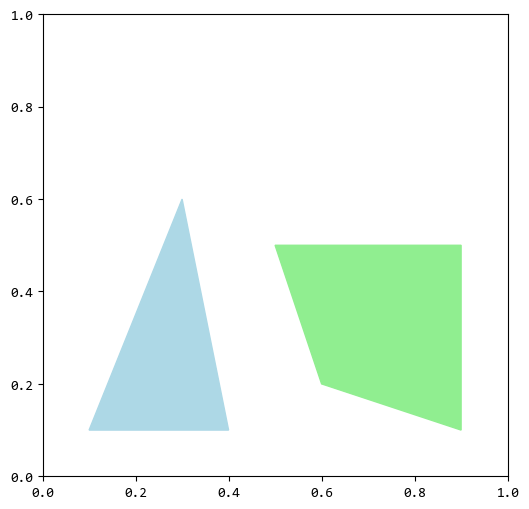## 1.3. 正多边形

matplotlib提供了专门绘制正多边形的对象CirclePolygon

fig = plt.figure(figsize=[6, 6])

p1 = mptch.CirclePolygon(xy=(0.3, 0.3),
resolution=6,
color='lightgreen')
p2 = mptch.CirclePolygon(xy=(0.6, 0.6),
resolution=8,
color='lightblue')

plt.show()


CirclePolygon对象的主要参数

1. xy：正多边形的中心点坐标
3. resolution：正多边形的边数
4. color：正多边形的颜色，默认蓝色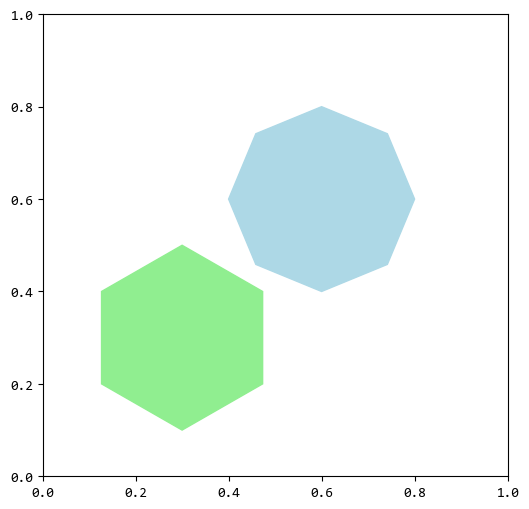# 2. 圆形类

## 2.1. 圆和椭圆

fig = plt.figure(figsize=[6, 6])

c = mptch.Circle(xy=(0.5, 0.5),
color="lightblue")
e = mptch.Ellipse(xy=(0.5, 0.5),
height=0.3,
width=0.4,
color="lightgreen")

plt.show()


Circle对象的主要参数

1. xy：圆心坐标
3. color：圆的颜色，默认蓝色

Ellipse对象的主要参数

1. xy：椭圆心坐标
2. height：椭圆的高度
3. width：椭圆的宽度
4. color：椭圆的颜色，默认蓝色

**PS. **当椭圆的heightwidth设置一样时，椭圆就是圆了。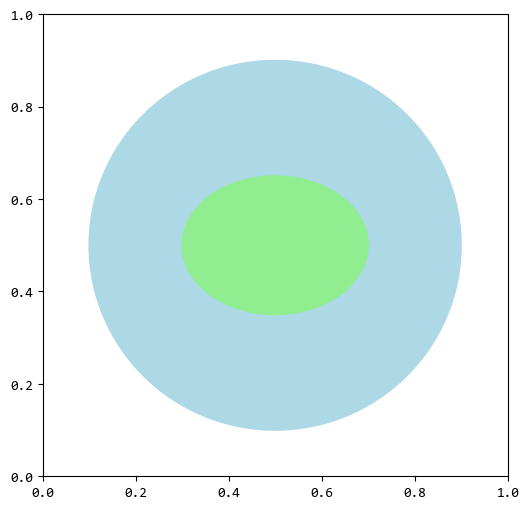# 3. 圆弧和扇形

fig = plt.figure(figsize=[6, 6])

#圆弧
a = mptch.Arc(xy=(0.4, 0.7),
width=0.5, height=0.5,
angle=270,
theta1=0, theta2=120,
linewidth=10,
color="lightblue")

#扇形
w = mptch.Wedge(center=(0.3, 0.5),
r=0.2,
theta1=30, theta2=330,
color="lightgreen")

plt.show()


Arc对象的主要参数

1. xy：圆弧的圆心坐标
2. width：圆弧的宽度
3. height：圆弧的高度
4. angle：圆弧朝向的角度，逆时针旋转
5. theta1：圆弧开始的角度，逆时针旋转
6. theta2：圆弧结束的角度，逆时针旋转
7. linewidth：圆弧的粗细
8. color：圆弧的颜色，默认蓝色

PS. widthheight相等时，圆弧相当于是的一段，不相等时，圆弧相当于是椭圆的一段。

Wedge对象的主要参数

1. xy：扇形的圆心坐标
2. r：扇形的半径
3. theta1：扇形开始的角度，逆时针旋转
4. theta2：扇形结束的角度，逆时针旋转
5. color：扇形的颜色，默认蓝色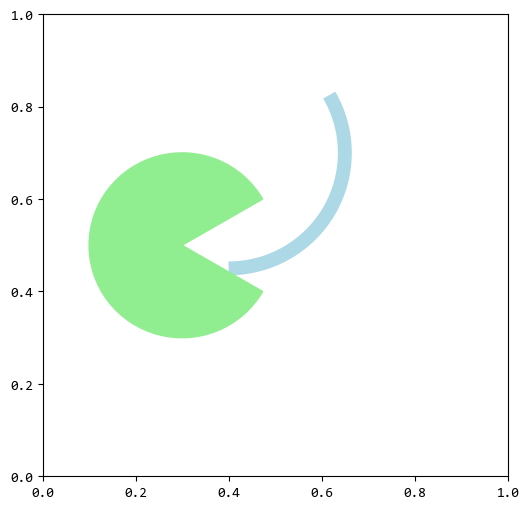# 4. 箭头

matplotlib中用Arrow对象来绘制箭头。

fig = plt.figure(figsize=[6, 6])

x, y, dx, dy  = 0.1, 0.2, 0.2, 0.2
a1 = mptch.Arrow(x=x, y=y,
dx=dx, dy=dy,
width=0.2, color="lightblue")
a2 = mptch.Arrow(x=x+dx, y=y+dy,
dx=dx, dy=dy,
width=0.4, color="lightgreen")

plt.show()


Arrow对象的主要参数

1. x：箭头的起点X坐标
2. y：箭头的起点Y坐标
3. dx：箭头的终点X坐标偏移起点X坐标的值
4. dy：箭头的终点Y坐标偏移起点Y坐标的值
5. width：箭头的宽度
6. color：箭头的颜色，默认蓝色

PS. 其实就是起点坐标(x, y)终点坐标(x+dx, y+dy)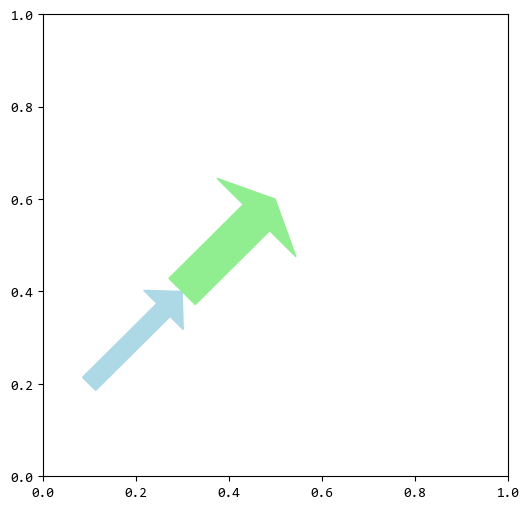# 5. 总结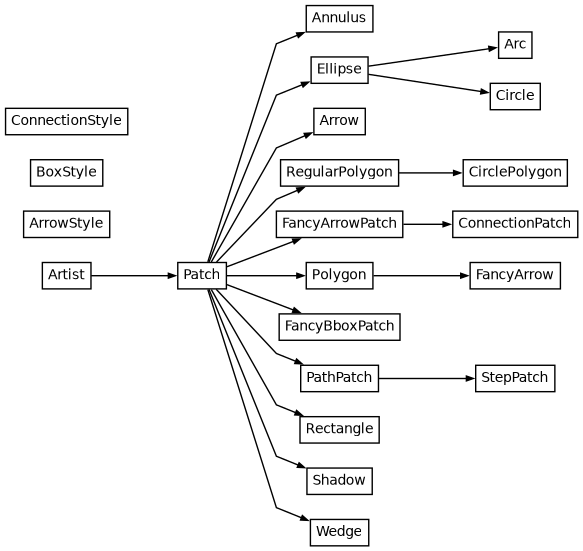posted @ 2023-09-19 10:32  wang_yb  阅读(156)  评论(3编辑  收藏  举报